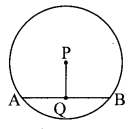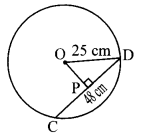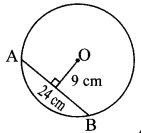# Maharashtra Board Class 8 Maths Solutions Chapter 17 Circle: Chord and Arc Practice Set 17.1

## Maharashtra State Board Class 8 Maths Solutions Chapter 17 Circle: Chord and Arc Practice Set 17.1

Question 1.
In a circle with centre P, chord AB is drawn of length 13 cm, seg PQ ⊥ chord AB, then find l(QB)Solution:
seg PQ ⊥ chord AB … [Given]
∴l(QB) = $$\frac { 1 }{ 2 }$$ l(AB)… [Perpendicular drawn from the centre of a circle to its chord bisects the chord]
∴l(QB) = $$\frac { 1 }{ 2 }$$ x 13 …[∵ l(AB) = 13 cm]
∴l(QB) = 6.5 cm

Question 2.
Radius of a circle with centre O is 25 cm. Find the distance of a chord from the centre if length of the chord is 48 cm.Solution:
seg OP ⊥ chord CD … [Given]
∴l(PD) = $$\frac { 1 }{ 2 }$$ l(CD) … [Perpendicular drawn from the centre of a circle to its chord bisects the chord]
∴l(PD) = $$\frac { 1 }{ 2 }$$ x 48 …[∵ l(CD) = 48 cm]
∴l(PD) = 24 cm …(i)
In ∆OPD, m∠OPD = 90°
∴[l(OD)]² = [l(OP)]² + [l(PD)]² … [Pythagoras theorem]
∴(25)² = [l(OP)]² + (24)² … [From (i) and l(OD) = 25 cm]
∴(25)² – (24)² = [l(OP)]²
∴(25 + 24) (25 – 24) = [l(OP)]² …[∵ a² – b² = (a + b) (a – b)]
∴49 x 1 = [l(OP)]²
∴[l(OP)]² = 49
∴l(OP) = √49 …[Taking square root of both sides]
∴l(OP) = 7 cm
∴The distance of the chord from the centre of the circle is 7 cm.

Question 3.
O is centre of the circle. Find the length of radius, if the chord of length 24 cm is at a distance of 9 cm from the centre of theSolution:
Let seg OP ⊥ chord AB
∴ l(AP) = $$\frac { 1 }{ 2 }$$ l(AB) … [Perpendicular drawn from the centre of a circle to its chord bisects the chord]∴l(AP) = $$\frac { 1 }{ 2 }$$ x 24 …[∵ l(AB) = 24 cm]
∴l(AP) = 12 cm …(i)
In ∆OPA, m∠OPA = 90°
∴[l(AO)]² = [l(OP)]² + [l(AP)]² … [Pythagoras theorem]
∴[l(AO)]² = (9)² + (12)² … [From (i) and l(OP) = 9 cm]
= 81 + 144
∴[l(AO)]² = 225
∴l(AO) = √225 …[Taking square root of both sides]
∴l(AO) = 15 cm
∴The length of radius of the circle is 15 cm.

Question 4.
C is the centre of the circle whose radius is 10 cm. Find the distance of the chord from the centre if the length of the chord is 12 cm.
Solution:
Let seg AB be the chord of the circle with centre C.
Draw seg CD ⊥ chord AB.∴l(AD) = $$\frac { 1 }{ 2 }$$ l(AB) …[Perpendicular drawn from the centre of a circle to its chord bisects the chord]
= $$\frac { 1 }{ 2 }$$ x 12 …[∵ l(AB) = 12 cm]
∴[l(AC)]² = [l(AD)]² + [l(CD)]² … [Pythagoras theorem]
∴(10)² = (6)² + [l(CD)]² … [From (i) and l(AC) = 10 cm]
∴(10)² – (6)² = [l(CD)]²
∴100 – 36 = [l(CD)]²
∴64 = [l(CD)]²
i. e. [l(CD)]² = 64
∴l(CD) = √64 …[Taking square root of both sides]
∴l(CD) = 8 cm
∴The distance of the chord from the centre of the circle is 8 cm.

Maharashtra Board Class 8 Maths Chapter 17 Circle: Chord and Arc Practice Set 17.1 Intext Questions and Activities

Question 1.
In the given figure, O is the centre of the circle. With reference to the figure fill in the blanks. (Textbook pg. No. 114)Solution:

1. Seg OD is radius of the circle.
2. Seg AB is diameter of the circle.
3. Seg PQ is chord of the circle.
4. ∠DOB is the central angle.
5. Minor arc : arc AXD, arc BD, arc AP, arc PQ, arc BQ, etc.
6. Major arc : arc PAB, arc PDQ, arc PDB, arc ADQ, etc.
7. Semicircular arc : arc ADB, arc AQB.
8. m (arc DB) = m∠DOB
9. m (arc DAB) = 360° – m∠DOB

Question 2.
Draw chord AB of a circle with centre O. Draw perpendicular OP to chord AB. Measure seg AP and seg PB. What do you observe. (Textbook pg. no. 114)
Solution:
l(AP) = l(PB) = 0.9 cm
∴the perpendicular drawn from the centre of the circle to its chord bisects the chord.Question 3.
Draw five circles with different radii. Draw a chord and perpendicular from the centre to each chord in each circle. Verify with a divider that the two parts of the chords are equal. (Textbook pg. no. 114)
Solution:
[Students should attempt the above activities on their own.]

Question 4.
Draw five circles of different radii on a paper. Draw a chord in each circle. Find the midpoint of each chord. Join the centre of the circle and midpoint of the chord as shown in the figure. Name the chord as AB and midpoint of the chord as P. Check with set-square or protractor that ∠APO or ∠BPO are right angles.
Check whether the same result is observed for the chord of each circle. (Textbook pg, no. 115)
Solution:
[Students should attempt the above activities on their own.]